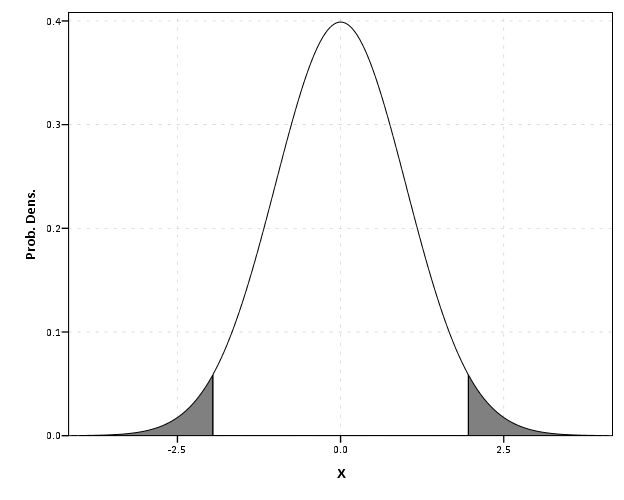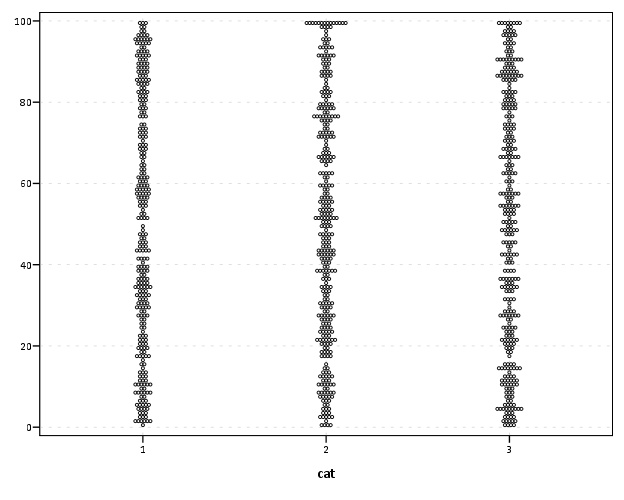# Some random SPSS graph tips: shading areas under curves and using dodging in binned dot plots

This is just a quick post on some random graphing examples you can do with SPSS through inline GPL statements, but are not possible through the GUI dialog. These also take knowing alittle bit about the grammar of graphics, and the nuts and bolts of SPSS’s implementation. First up, shading under a curve.

I assume the motivation for doing this is obvious, but it is alittle advanced GPL to figure out how to accomplish. I swore someone asked how to do this the other day on NABBLE, but I could not find any such questions. Below is an example.

``````*****************************************.
input program.
loop #i = 1 to 2000.
compute X = (#i - 1000)/250.
compute PDF = PDF.NORMAL(X,0,1).
compute CDF = CDF.NORMAL(X,0,1).
end case.
end loop.
end file.
end input program.
dataset name sim.
exe.

formats PDF X (F2.1).

*area under entire curve.
GGRAPH
/GRAPHDATASET NAME="graphdataset" VARIABLES=X PDF MISSING=LISTWISE
REPORTMISSING=NO
/GRAPHSPEC SOURCE=INLINE.
BEGIN GPL
SOURCE: s=userSource(id("graphdataset"))
DATA: X=col(source(s), name("X"))
DATA: PDF=col(source(s), name("PDF"))
GUIDE: axis(dim(1), label("X"))
GUIDE: axis(dim(2), label("Prob. Dens."))
ELEMENT: area(position(X*PDF), missing.wings())
END GPL.

*Mark off different areas.
compute tails = 0.
if CDF <= .025 tails = 1.
if CDF >= .975 tails = 2.
exe.

*Area with particular locations highlighted.
GGRAPH
/GRAPHDATASET NAME="graphdataset" VARIABLES=X PDF tails
/GRAPHSPEC SOURCE=INLINE.
BEGIN GPL
SOURCE: s=userSource(id("graphdataset"))
DATA: X=col(source(s), name("X"))
DATA: PDF=col(source(s), name("PDF"))
DATA: tails=col(source(s), name("tails"), unit.category())
SCALE: cat(aesthetic(aesthetic.color.interior), map(("0",color.white),("1",color.grey),("2",color.grey)))
SCALE: cat(aesthetic(aesthetic.transparency.interior), map(("0",transparency."1"),("1",transparency."0"),("2",transparency."0")))
GUIDE: axis(dim(1), label("X"))
GUIDE: axis(dim(2), label("Prob. Dens."))
GUIDE: legend(aesthetic(aesthetic.color.interior), null())
GUIDE: legend(aesthetic(aesthetic.transparency.interior), null())
ELEMENT: area(position(X*PDF), color.interior(tails), transparency.interior(tails))
END GPL.
*****************************************.``````The area under the entire curve is pretty simple code, and can be accomplished through the GUI. The shading under different sections though requires a bit more thought. If you want both the upper and lower tails colored of the PDF, you need to specify seperate categories for them, otherwise they will connect at the bottom of the graph. Then you need to map the categories to specific colors, and if you want to be able to see the gridlines behind the central area you need to make the center area transparent. Note I also omit the legend, as I assume it will be obvious what the graph represents given other context or textual summaries.

# Binning scale axis to produce dodging

The second example is based on the fact that for SPSS to utilize the dodge collision modifier, one needs a categorical axis. What if you want the axis to really be scale though? You can make the data categorical but the axis on a continuous scale by specifying a binned scale, but just make the binning small enough to suit your actual data values. This is easy to show with a categorical dot plot. If you can, IMO it is better to use dodging than jittering, and below is a perfect example. If you run the first GGRAPH statement, you will see the points aren’t dodged, although the graph is generated just fine and dandy with no error messages. The second graph bins the X variable (which is on the second dimension) with intervals of width 1. This ends up being exactly the same as the continuous axis, because the values are all positive integers anyway.

``````*****************************************.
set seed = 10.
input program.
loop #i = 1 to 1001.
compute X = TRUNC(RV.UNIFORM(0,101)).
compute cat = TRUNC(RV.UNIFORM(1,4)).
end case.
end loop.
end file.
end input program.
dataset name sim.

GGRAPH
/GRAPHDATASET NAME="graphdataset" VARIABLES=X cat
/GRAPHSPEC SOURCE=INLINE.
BEGIN GPL
SOURCE: s=userSource(id("graphdataset"))
DATA: X=col(source(s), name("X"))
DATA: cat=col(source(s), name("cat"), unit.category())
COORD: rect(dim(1,2))
GUIDE: axis(dim(1), label("cat"))
ELEMENT: point.dodge.symmetric(position(cat*X))
END GPL.

*Now lets try to bin X so the points actually dodge!.
GGRAPH
/GRAPHDATASET NAME="graphdataset" VARIABLES=X cat
/GRAPHSPEC SOURCE=INLINE.
BEGIN GPL
SOURCE: s=userSource(id("graphdataset"))
DATA: X=col(source(s), name("X"))
DATA: cat=col(source(s), name("cat"), unit.category())
COORD: rect(dim(1,2))
GUIDE: axis(dim(1), label("cat"))
ELEMENT: point.dodge.symmetric(position(bin.rect(cat*X, dim(2), binWidth(1))))
END GPL.
****************************************.``````Both examples shown here only take slight alterations to code generatable through the GUI, but take a bit more understanding of the grammar to know how to accomplish (or even know they are possible). You unfortunately can’t implement Wilkinson’s (1999) true dot plot technique like this (he doesn’t suggest binning, but by choosing where the dots are placed by KDE estimation). But this should be sufficient for most circumstances.

1.#### Reece

/  March 19, 2013

Awesome review on shading. Do you know of an easy way to shade between two curves instead of shading to the x axis as you do in this post? I know the chart builder has the built in difference graph type, but the syntax is hard to make sense of and it seems to limit what else you can do on the chart.

The best I’ve come up with is doing it in Illustrator. I hate having to work up charts in a graphics program.

•#### apwheele

/  March 19, 2013

Sure, here is a brief example. You would use area.difference to accomplish that.

``````********************************************************************.
data list free / x low high middle.
begin data
1 1 3 2
2 2 4 3
3 3 5 4
4 4 6 5
5 5 7 6
end data.
dataset name ex.
dataset activate ex.

GGRAPH
/GRAPHDATASET NAME="graphdataset" VARIABLES=x low high middle
MISSING = VARIABLEWISE
/GRAPHSPEC SOURCE=INLINE.
BEGIN GPL
SOURCE: s=userSource(id("graphdataset"))
DATA: x=col(source(s), name("x"))
DATA: middle=col(source(s), name("middle"))
DATA: low=col(source(s), name("low"))
DATA: high=col(source(s), name("high"))
COORD: rect(dim(1,2))
GUIDE: axis(dim(1), label("x"))
GUIDE: axis(dim(2), label("Value"))
transparency.exterior(transparency."1"), color.interior(color.grey))
ELEMENT: line(position(x*middle), color.interior(color.black))
END GPL.
********************************************************************.``````

The GUI can aid to a certain extent to help figure out the graph algebra, but sometimes it is pretty verbose and difficult to parse.

•#### Reece

/  March 26, 2013

That was just what I needed to figure it out. An update: using your method causes some problems if there is a splitting variable (e.g., mapped to color), especially if there are >2 values of that variable. The problem was with area.difference, which isn’t necessary if there are already variables for the upper/lower bounds of the shaded region. I found this syntax:

to work great. I guess the area.difference element is designed to compute the difference area in the GGRAPH procedure rather than using pre-supplied minima and maxima.

2.#### apwheele

/  March 26, 2013

Thanks for the feedback Reece. I have had inconsistent results when using area.difference with multiple groups as well recently (I was kind of hoping it was due to my older V15 though, and was fixed in newer versions!)

A (annoying) solution to make multiple areas is to reshape the data long to wide, and then make a separate element statement for every group.